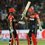# Arithmetic Mean!

The arithmetic mean of a number of pairwise distinct prime numbers is 27. Determine the biggest prime among them.Note by Ayush G Rai
4 years, 4 months ago

This discussion board is a place to discuss our Daily Challenges and the math and science related to those challenges. Explanations are more than just a solution — they should explain the steps and thinking strategies that you used to obtain the solution. Comments should further the discussion of math and science.

When posting on Brilliant:

• Use the emojis to react to an explanation, whether you're congratulating a job well done , or just really confused .
• Ask specific questions about the challenge or the steps in somebody's explanation. Well-posed questions can add a lot to the discussion, but posting "I don't understand!" doesn't help anyone.
• Try to contribute something new to the discussion, whether it is an extension, generalization or other idea related to the challenge.

MarkdownAppears as
*italics* or _italics_ italics
**bold** or __bold__ bold
- bulleted- list
• bulleted
• list
1. numbered2. list
1. numbered
2. list
Note: you must add a full line of space before and after lists for them to show up correctly
paragraph 1paragraph 2

paragraph 1

paragraph 2

[example link](https://brilliant.org)example link
> This is a quote
This is a quote
    # I indented these lines
# 4 spaces, and now they show
# up as a code block.

print "hello world"
# I indented these lines
# 4 spaces, and now they show
# up as a code block.

print "hello world"
MathAppears as
Remember to wrap math in $$ ... $$ or $ ... $ to ensure proper formatting.
2 \times 3 $2 \times 3$
2^{34} $2^{34}$
a_{i-1} $a_{i-1}$
\frac{2}{3} $\frac{2}{3}$
\sqrt{2} $\sqrt{2}$
\sum_{i=1}^3 $\sum_{i=1}^3$
\sin \theta $\sin \theta$
\boxed{123} $\boxed{123}$

Sort by:

This problem came in NMTC 2015. The prime numbers must be less than 54. Listing first few possibilities 53 and 1, 52 and 2, 51 and 3, 50 and 4, 49 and 5, 48 and 6, 47 and 7. Only the pair 47 and 7 are both primes and 47 is the biggest.

- 4 years, 4 months ago

you are absolutely correct.Did you appear for the NMTC 2nd level test?

- 4 years, 4 months ago

- 4 years, 4 months ago

Yes! It was really tough for me.

- 4 years, 4 months ago

Can solve the other set of problems?their also from NMTC.

- 4 years, 4 months ago

You seem to be assuming there are only two prime numbers, when the question doesn't explicitly state that.

Otherwise, there can be other solutions like $(3,7,71)$

- 4 years, 4 months ago

It clearly states pair wise that means only two prime numbers.

- 4 years, 4 months ago

Nope. Pair wise distinct does not mean only two. Pair wise distinct means that given a set of elements, no two elements (pair) of the set are the same. It does not restrict the number of elements in the set.

https://proofwiki.org/wiki/Definition:Distinct/Plural/Pairwise_Distinct

- 4 years, 4 months ago

definition:A set of objects is pairwise distinct if each pair of elements of that set is distinct.
It clearly states a pair of elements which means two elements.

- 4 years, 4 months ago

each pair. Not a pair.

- 4 years, 4 months ago

that makes no difference.

- 4 years, 4 months ago

You can check the top comment here or this. Notice how none of them refer to only two elements when talking about pairwise distinct elements.

- 4 years, 4 months ago

- 4 years, 4 months ago

I still don't agree with you.

- 4 years, 4 months ago

Sure. No problem.

- 4 years, 4 months ago